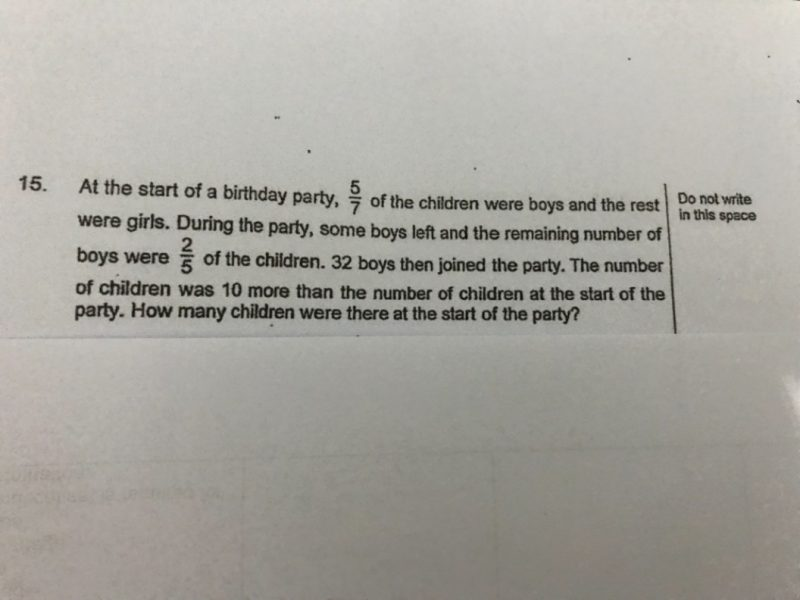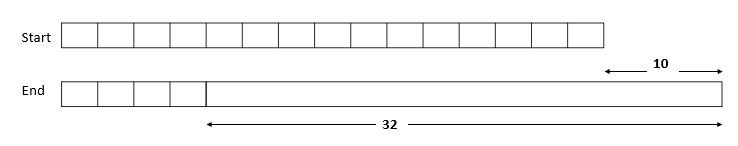# QuestionCan anyone able to show how to draw model drawing?

ThanksBoys Girls 5 2 -X 2 3 +32

Number of girls is the same, so modify the table to be

 Boys Girls x 3 15 6 -X x 2 4 6 + 32

Total number of children is increased by 10, which is due to the movement of boys only. So, we can draw the above model for boys. From model,

11U = 32 – 10

11U = 22

1U  = 2.

Boys at start = 15U = 15 x 2 = 30.

Girls at start = 6U   = 6 x 2  = 12

Total children at start = 30 + 12 = 42

Verify

 Boys Girls Total 30 12 42 -22 8 12 +32 40 12 52

52 – 42 = 10.

0 Replies 0 Likes